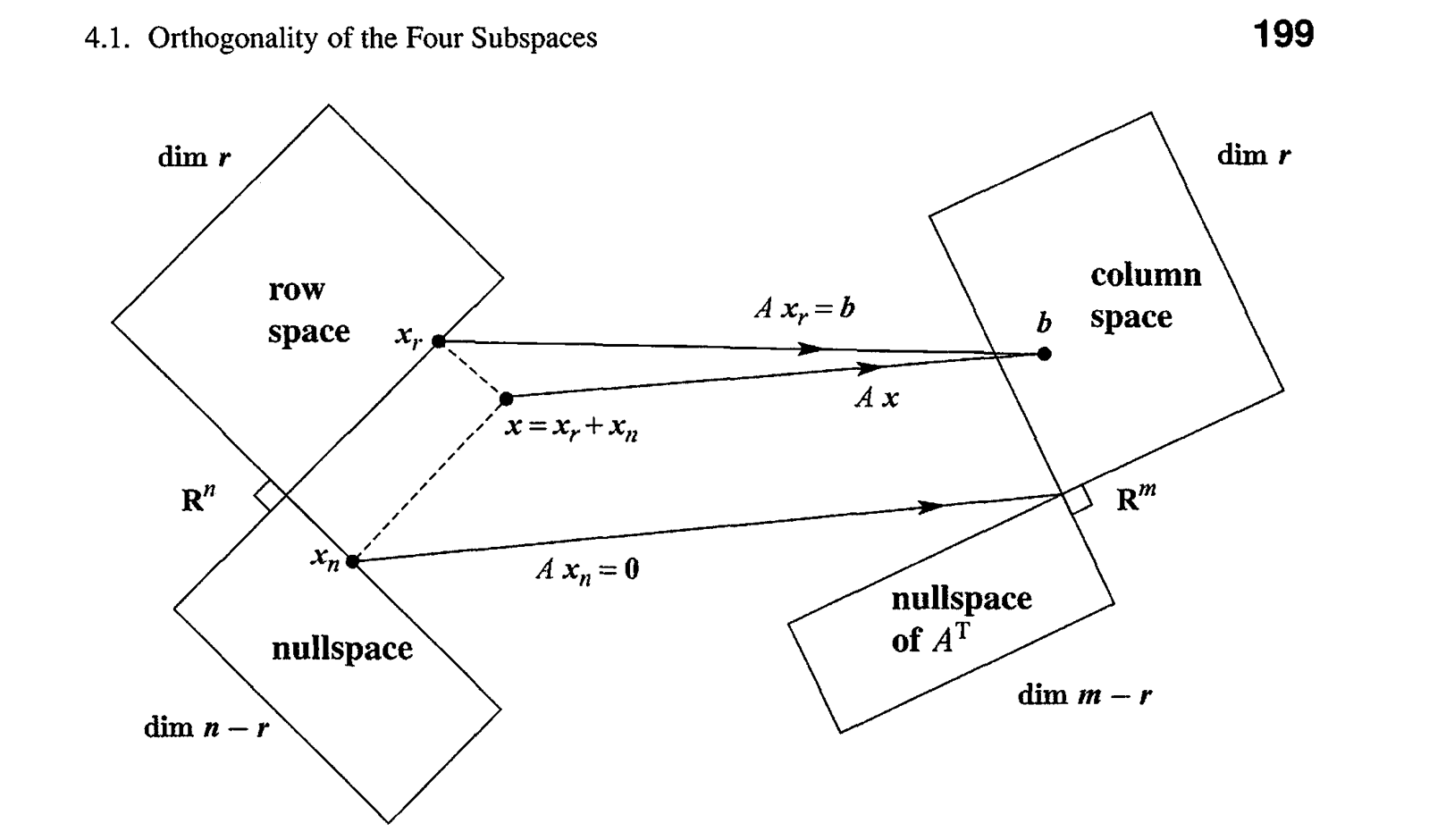## Eigenvalues and Eigenvectors

### 6.1 Introduction

certain vectors x are in the same direction as Ax

basic equation:

​ $Ax = \lambda x$

how to compute:

• let $det(A-\lambda x)= 0$ ,find the roots == find eigenvalues, find eigenvectors in Null space

$A^nx = \lambda^n x$

span eigenspace

Projection: $\lambda = 1$ or 0

Reflection: $\lambda = 1$ or -1

Rotation: complex eigenvalues only

product of eigenvalues == determinant == product of pivots

sum of eigenvalues == sum of diagonal entries ( not pivots ) == trace

### 6.2 Diagonalizing

eigenvectors in columns of S, eigenvalues in diagonal of Λ

$Λ = S^{-1}AS$

$A = SΛS^{-1}$

Independent x from different λ:

• $c_1 λ_1 x_1 + c_2 λ_2 x_2 = 0$
• $c_1 λ_2 x_2 + c_2 λ_2 x_2 = 0$

subtract

• $(λ_1 - λ_2)c_1x_1 = 0$

diagonalizability:

• enough eigenvectors (maybe same λ) so that S is invertible

$u_k = A^k u_0 = S \Lambda^k S^{-1}u_0$

1. $u_0 = c_1x_1 + c_2x_2 + .. +c_nx_n$ eigenvector basis

2. multiply $\lambda_i ^ k$

3. add up

A,B share same eigenvector matrix S if and only if AB = BA

?Heisenberg uncertainty principle

position matrix P, momentum matrix Q, $QP-PQ = I$

knew P still could not know Q

$|Px||Qx| \ge \frac{1}{2}|x|^2$

### 6.4 Symmetric Matrices

1. real eigenvalues
2. orthonormal eigenvectors

Spectral Theorem

(principle of axis theorem)

$A = Q\Lambda Q^T$

Normal Matrices $\bar A^TA = A \bar A^T$

symmetric, skewed-symmetric, orthogonal

A has n orthonormal vectors ($A = Q\Lambda \bar Q ^T$) if and only if A is normal

Real Eigenvalues

proof:

• $Ax = \lambda x$ $\bar x ^TA = \bar x ^T \bar\lambda$ ( $A = A^T$ )( conjugate and transpose )
• $\bar x^T A x = \bar x^T \lambda x$ $\bar x ^ T A x = \bar x ^ T \bar\lambda x$

left side the same

therefor $\lambda == \bar \lambda$

Orthonormal

proof:

• no eigenvalues repeated
• Allow repeated eigenvalues ( ? Schur’s Theorem )

sum of rank one projection matrices

$A = \lambda_1x_1x_1^T + \lambda_2x_2x_2^T+…$

$= \lambda_1P_1 +\lambda_2P2+….$

number of positive pivots == number of positive eigenvalues

$A = LDL^T$

### 6.5 Positive Definite Matrices

All λ > 0

quick way to test

1. All pivots positive

2. n eigenvalues positive

3. $x^TAx$ is positive except x = 0

4. $A == R^TR$ (symmetric) and R has independent columns ($x^T R^TRX >= 0$)

5. n upper left determinants

R can be chosen:

• rectangular / $(L\sqrt D)^T$ / $Q \sqrt\Lambda Q^T$

$x^TAx$

• (2*2) = $ax^2 + 2bxy + cy^2 > 0$ ( ellipse $z = x^2/a^2 + y^2/b^2$ )

Application

1. tilted ellipse $x^TAx$
2. lined - up ellipse $X^T\Lambda X = 1$
3. rotation matrix Q

axes: eigenvectors

half-length: $1/\sqrt\lambda$

### 6.6 Similar Matrices

DEF: A similar to B (A family)

• $B = M^{-1}AM$

Property:

• A and B have same eigenvalues
• x a eigenvector of A and $M^{-1}x$ eigenvector of B

Jordan Form

• triple eigenvalues while one eigenvector
• J with λ in the diagonal and 1 above
• similar to every matrices with repeated eigenvalues λ and one eigenvector
• λ repeated only once than J == Λ
• Jordan Block
• make A as simple as possible while preserving essential properties

### 6.7 Singular Value Decomposition

SUMMARY

$A = U \Sigma V^T$

$\Sigma^2 = \Lambda$ (of $A^TA$ and $AA^T$ )

$U = Q$ (of $AA^T$ in $R^m$)

$V = Q$ (of $A^TA$ in $R^n$)

orthonormal basis of row space {$v_1, v_2, … v_r$}

orthonormal basis of null space{ $v_{r+1}, v_{r+2},…v_n$}

orthonormal basis of column space{ $u_1, u_2,…u_r$}

orthonormal basis of left null space { $u_{r+1}, u_{r+2}, … u_m$}

Rotation – Stretch – Rotation$Av_1 = \sigma_1u_1$ …

so $AV = U\Sigma$

(m n) (n n) = (m m) (m n)

$V$ and $U$ are orthogonal matrices

$\Sigma$ = ( old r*r $\Sigma$ ) + (m-r zero rows) + ( n-r zero columns )

therefore …

when A positive definite symmetric

$A = U \Sigma V^T = Q\Lambda Q^{T}$

### 7.3 Diagonalization and the Pseudoinverse

change bases

$\Lambda_{ w - w } = S^{-1}{std - w} A{std} S_{ w - std }$

$\Sigma_{ v - u } = U^{-1}{std - u} A{std}V_{v - std}$

Polar Decomposition

orthogonal and semidefinite

rotation and stretching

$A = U\Sigma V^T = ( UV^T ) (V \Sigma V^T) = QH$

Pseudoinverse

$A^+ = V\Sigma^+ U^T$